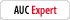# \$0.00 not \$-20.00....

Tbrasch
Registered: Oct 21 2008
Posts: 17

Well i've tried creating a formula that stops the number at zero and not go negitive.
i first tried it by going to the value range and setting it to zero.. but then i get
an error message that pops up.. and that isn't ok.

i then wrote out a formula to try to work it all out for me.. but i dont think i did it right.

if(this.getField("Amount 9Net Amt Due DEA BA").value<0)this.getField("Amount 9Net Amt Due DEA BA").value=0

i also have zero training on writing script... so yea i understand if it is totally wrong.

Any Ideas?

My Product Information:
Acrobat Pro 9.0, Windows
George_JohnsonRegistered: Jul 6 2008
Posts: 1875
You need to provide more information about how your form is set up. In particular, describe how this particular field is used. For example, is it a calculated field, a field that a user can make an entry, etc.

George
Tbrasch
Registered: Oct 21 2008
Posts: 17
The form is an employee expence report form. There are four fields involved, one is the sub total of the form, then a space if the employee received a cash advance. The cash advance is subtracted from the sub total. If the number is positive, the amount needs to fill in to the area for amount due employee. If the number is negative the amount needs to fill into the field for the amount due the company. Neither number however, should appear with a leading negative notation.

Thank you
George_JohnsonRegistered: Jul 6 2008
Posts: 1875
OK. One thing you can do is set up a custom calculation script for the amount due employee field and the amount due company field. They could look something like:

`// Custom calculation script for amount due employee field // Get field values, as numbersvar v1 = +getField("subtotal").value;var v2 = +getField("cash_advance").value;var diff = v1 - v2; // Set the value of this field based on the differenceif (diff > 0) {event.value = diff;} else {event.value = "";}`

`// Custom calculation script for amount due company field // Get field values, as numbersvar v1 = +getField("subtotal").value;var v2 = +getField("cash_advance").value;var diff = v1 - v2; // Set the value of this field based on the differenceif (diff < 0) {event.value = -diff;} else {event.value = "";}`

You'll have to change the field names in the code above to match yours, but it should get you started.

George
Tbrasch
Registered: Oct 21 2008
Posts: 17
Thank you so much!